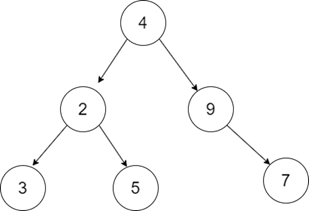# Binary Tree Tilt in C++

Let us consider that we have the root node of a binary tree; the task is to find and return the sum of tilt of every node.

The tilt of a binary tree is nothing but constructing the binary tree by finding the absolute difference of child nodes in the left subtree and the right subtree in each level. At some particular level, the nodes which don't have any child nodes, we simply tilt by replacing that node with zero.

Example

InputOutput: 15

Explanation: Finding the tilt at every level of the given binary tree,

The tilt of node 3 = 0

The tilt of node 5 = 0

The tilt of node 7 = 0

The tilt of node 2 = abs(3-5)= 2

The tilt of node 9 = abs(0-7)= 7

The tilt of node 4 = abs((3+5+2)- (9+7))= 6

The sum of tilt of all the nodes is = 2+7+6= 15

## Approach to Solve this Problem

The simple approach to solve this particular problem is to use the Post Order Breadth First Search traversal.

While traversing the binary tree, we will try to find the sum of all the nodes of its left subtree and then the right subtree. Once the sum is found, then we will find the tilt of all the nodes by calculating the absolute difference of its left sum and right sum of subtrees.

• Take a binary tree, which will be the input.
• An integer function sumNodes(treenode*node) takes the root node of the tree and returns the sum of the left subtree and the right subtree.
• An integer function findTilt(treenode*root) takes the root node as the input parameter and returns the sum of tilt of all the nodes.

## Example

Live Demo

#include<iostream>
using namespace std;
struct treenode {
int data;
treenode * left;
treenode * right;
};
struct treenode * createNode(int d) {
struct treenode * root = new treenode;
root -> data = d;
root -> left = NULL;
root -> right = NULL;
return root;
}
int sumNodes(treenode * root, int &amp; sum) {
if (root == NULL) return 0;
int lsum = sumNodes(root -> left, sum);
int rsum = sumNodes(root -> right, sum);
sum += abs(lsum - rsum);
return lsum + rsum + root -> data;
}
int findTilt(treenode * root) {
int sum = 0;
if (root == NULL) {
return 0;
}
sumNodes(root, sum);
return sum;
}
int main() {
struct treenode * root = NULL;
root = createNode(4);
root -> left = createNode(2);
root -> right = createNode(9);
root -> left -> right = createNode(5);
root -> left -> left = createNode(3);
root -> right -> right = createNode(7);
cout << findTilt(root) << endl;
return 0;
}

Running the above code will generate the output as,

## Output

15

In the given binary tree, the sum of tilt of all the nodes at every level of the tree is 15.